Innovation for Every Engineer.
HomeBlog

# Modeling and Control of Full Bridge Push-Pull Bi-Directional DC/DC Converter

Summary

Full bridge push-pull bi-directional DC/DC converters are mostly modeled by state space averaging method with the complex modeling process. Taking the isolated form push-pull bi-directional DC/DC converter as the research object, this article adopted the pulse-width modulation switch model method to obtain the equivalent circuit model of this converter. Based on this model, this article constructed a closedloop control system of the converter under different working modes, carried out the design and verification of voltage control loop and realized the constant voltage charge-discharge control strategy. The experimental and simulation results verify the correctness of the conclusion.

 Core Full bridge push-pull Purpose To obtain the equivalent circuit model of the converter English name Bidirectional DC/DC converters Category Electromechanical device Function Converting a source of direct current (DC) from one voltage level to another Feature Adopting the pulse-width modulation switch model method

Catalogs

 Catalogs 1. Foreword 2.2 Modeling of bi-directional DC/DC converter 4 Epilogue 2. Principle and design 2.3 Voltage Closed-loop Control and Simulation 2.1 The working principle of circuit 3 Experimental result

Introduction

1. Foreword

Bidirectional DC/DC converters have been widely applied in many fields such as electric vehicles and battery energy storage systems. Bidirectional DC/DC converters have different circuit topologies according to their applications.I have seen some examples of using Buck/Boost converter to realize the bi-directional flow of electric energy. Because there is no transformer in the converter, so the electrical isolation of the high and low voltage side can not be realized, and only a small voltage range has been allowed. In order to solve this problem, it is proposed to use isolation transformer in bidirectional full-bridge DC/DC transform circuit, but the converter uses too many switching devices and therefore causes too many power losses. The isolated full bridge push-pull bi-directional DC/DC converter has been widely used due to the advantages of both types of converters of itself.

At present, the state space averaging method is used to deduce the small signal model of bidirectional DC/DC converter, which needs a large amount of mathematical derivations and calculations. In order to simplify the modeling process, the full-bridge push-pull bi-directional DC/DC converter is modeled by using PWM-switch modeling method. The transfer function between output voltage and duty cycle of the converter is obtained in two modes: boost mode and buck mode. In addition , the voltage closed loop control system is constructed based on the derived circuit model, and the voltage loop is designed and corrected by the compensation network , realizing the constant voltage control of the full-bridge push-pull bidirectional DC/DC converter.

First step in developing feedback control for a dc-dc converter is modeling. Here, we model the buck converter in terms of average behavior.

Detail

2. Principle and design

2.1 The working principle of circuit

Figure 1 shows the topology of the main circuit of full bridge push-pull bi-directional DC/DC converter.

• ^The power switch tubes S1～S4  are arranged in the form of a full-bridge circuit;

• ^the power switch tubes S5～S6 are arranged in the form of a push-pull circuit;

• ^CHV is a parallel capacitor with HVDC busbar;

• ^CLV is a parallel capacitor with LVDC busbar;

• ^L is a  low-voltage-side energy storage filter inductor;

• ^HV is high-voltage-side DC bus;

• ^LV is a low-voltage-side DC bus.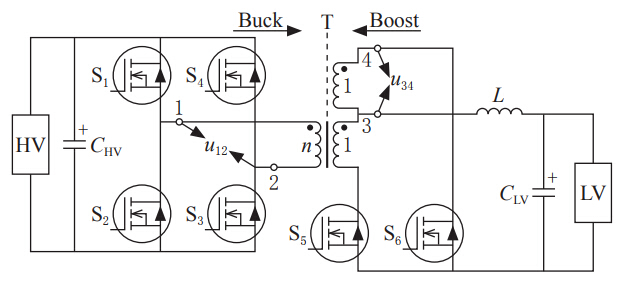Figure 1 Main circuit of bidirectional DC/DC converter

Figure 2 and 3 respectively give the schematic diagrams of the PWM driving waveform of the power switch tube working in boost and buck mode, and the schematic diagram of the voltage waveform of the primary and secondary sides of the transformer. The u12 shown in the diagram is the transformer primary-side voltage and the u34 is the transformer sub-side voltage.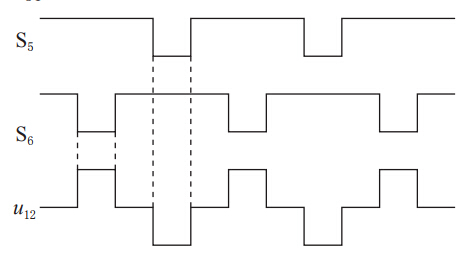Figure 2 Waveforms of the  power switch tube working in boost mode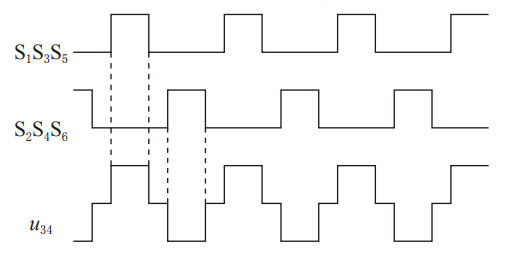Figure 3 Waveforms of the  power switch tube working in buck mode

2.2 Modeling of bidirectional DC/DC converter

The basic idea of PWM-switch modeling method: the nonlinear part will be linearized by taking an average value of the voltage and the current of it in one switching period when it is a stable circuit, and therefore the nonlinear circuit will turn into a linear circuit. The push-pull bidirectional DC/DC converter working in the buck mode can be equivalent to the circuit shown in Fig. 4 (the circuit in the dashed box is meant to indicate the nonlinear circuit), which turns on during [0, DTs] and turns off during [DTs, Ts], where Ts is the switching cycle and D is the duty ratio of the full bridge push-pull bi-directional DC/DC converter turn on.

In the mathematical expressions presented in this article, all the uppercase variables represent the steady-state values, and all the lowercase variables represent the instantaneous values.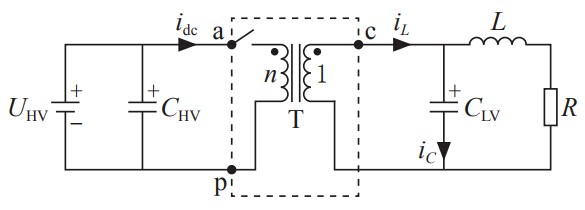Figure 4 Conducting circuit in buck mode operation

In the equivalent circuit shown in figure 4, the voltage and current are averaged during a PWM switching cycle, among which idc is primary instantaneous current and iL is secondary instantaneous current of transformer; iC is instantaneous current of electric capacity CLV; ucp is the instantaneous voltage between nodes c and p; uap is the instantaneous voltage between nodes a and p; n is the transformation ratio.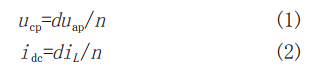Assuming that the duty cycle is d=D, now add an AC small signalto its value attachment (where small angle brackets denote AC small signals and the other variables following are the same), the complete instantaneous value expression for duty cycle is as shown in formula 3: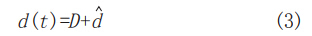Then substituting equation 3 into the original yields equation 1 and 2, so we have: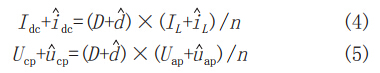Neglecting the product term of AC small signal, and equation 4 and 5 of  AC small signal can be simplified as equation 6 and 7: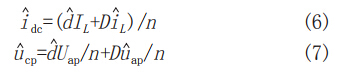The mathematical relations of voltage and current working in steady state (following yields equation 8 and 9) derived from original yields equation 1 and 2, along with equation 6 and 7 can be used to obtain equivalent circuit model of bidirectional DC/DC converter in Buck mode (see the two-port circuit shown in the dashed box of figure 5). The model is linear since the constraint equations describing the two-port circuit are all linear equations. It can be learned from the above modeling process that the linear model of the full bridge push-pull bidirectional DC/DC converter can be easily established by using the switching model modeling method, which has the advantages of less calculation and simple derivation. It can be learned from the above modeling process that the linear model of the full bridge push-pull bidirectional DC/DC converter can be easily established by using the PWM-switch modeling method, which has the advantages of less calculation and simple derivation.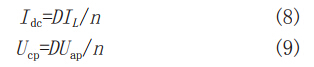The equation 10 gives transfer function between the output voltage and duty cycle of low voltage side bus according to laws of KCL and KVL.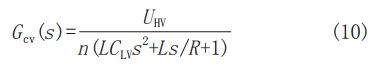Figure 5 gives the equivalent circuit of bidirectional DC/DC in Buck mode obtained from equation 10, among which UHV is the operating voltage of high voltage side bus in steady state, uLV is the instantaneous voltage of low voltage side bus, n is the transformer ratio, R is the load resistance of low-voltage-side DC bus,Uap/D can be regarded as controlled voltage source, andIL/n can be regarded as controlled current source.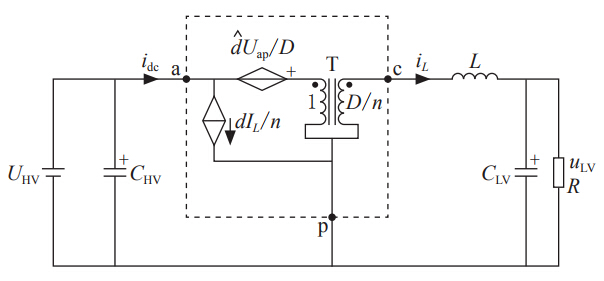Figure 5 Small signal equivalent circuit in Buck mode operation

The transfer function between duty cycle and output voltage in Boost mode can be obtained by using the same analysis method as Buck mode, which has shown in equation 11. R1 is the load of high voltage side bus.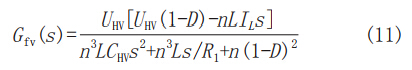2.3 Voltage Closed-loop Control and Simulation

Figure 6 gives the block diagram of the closed loop control system of small signal circuit model of the full bridge push-pull bidirectional DC/DC converter in two different operating modes. Charge and discharge at constant voltage in two modes can be realized by voltage closed-loop control.

What we can see from figure 6:

Uref is the given voltage of high-voltage-side and low-voltage-side DC bus; Hv(s) is the transfer function of sampling; Gm(s) is the transfer function of PWM; Gcv(s) is the transfer function of PI of voltage control loop; Gvd(s) is the transfer function of voltage versus duty cycle.Figure 6 Block diagram of voltage closed loop control

To theoretically verify the correctness of mathematical model establishment and the feasibility of voltage control strategy, we use the PSIM 9.0 software tool for simulation and analysis in this article. Figures 7 and 8 have respectively shown the simulated waveforms in different operating modes. In Buck mode operation, the voltage of low voltage bus can be stabilized at 12V, so the constant voltage charging has been realized; and in Boost mode operation, the voltage of high voltage bus can be stabilized at 400V, so the constant voltage discharge has been realized, which theoretically verifies the correctness of mathematical model establishment and the feasibility of voltage control strategy. u12 and u34 are the voltages of the primary and secondary sides of the transformer respectively, uHV and uLV are voltages of high-voltage side and low-voltage side bus.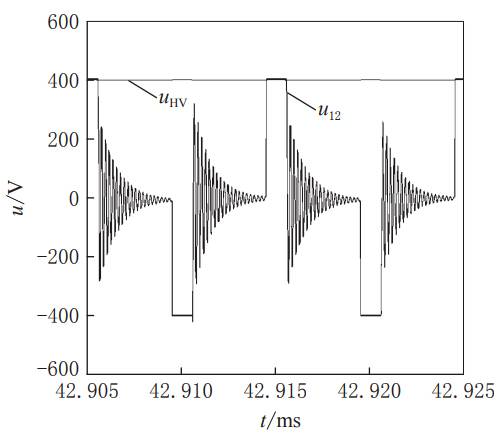Figure 7 Simulated voltage waveform in boost mode operation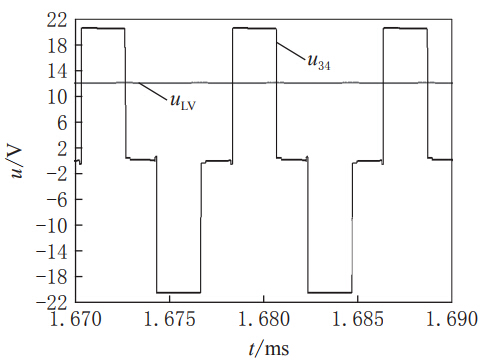Figure 8 Simulated voltage waveform in buck mode operation

Analysis

3 Experimental result

In order to verify the correctness of the small-signal circuit model and the feasibility of voltage feedback control, we built an experimental platform of full-bridge push-pull bi-directional DC/DC converter using TMS320F28035 as the core control chip.

Table 1 gives the main circuit parameters of the bidirectional DC/DC converter based on the full bridge push-pull circuit structure.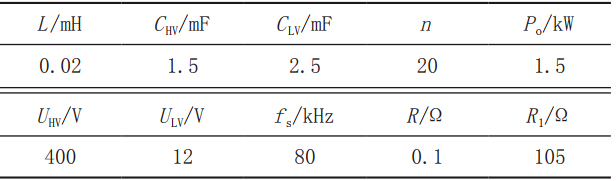Table 1 Basic circuit parameters of converter

Figure 9 gives the output voltage waveform of secondary voltage and low-voltage-side DC bus of transformer in buck mode operation when steady input voltage of high-voltage-side DC bus is 400V (provided by programmable DC power supply) and load resistance of low-voltage-side DC bus is 0.1Ω (provided by programmable DC electronic load). Here the output current of low-voltage-side DC bus iL is stable at about 120A, the output voltage uLV is about 12V, and the output power of low-voltage-side bus Po is about 1.5kW which meets the requirement of rated power designed, now we have realized  the constant-voltage charging of low-voltage-side DC bus.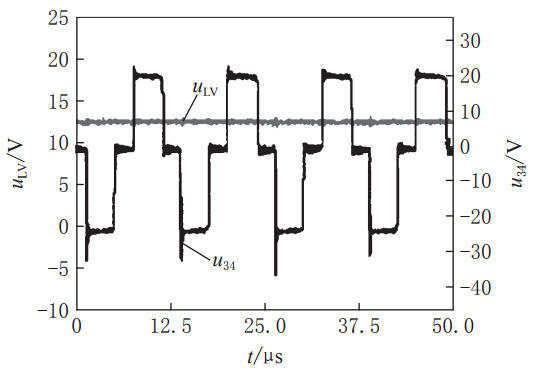Figure 9 The voltage waveform in Buck mode operation

Figure 10 gives the output voltage waveform of primary voltage and  high-voltage-side DC bus of transformer in boost mode operation when steady input voltage of low-voltage-side DC bus is 12V (provided by low-voltage DC power supply) and load resistance of high-voltage-side DC bus is 105Ω (provided by high voltage resistance load box). Here the output current of high-voltage-side DC bus iH is stable at about 3.7A, the output voltage of high-voltage-side DC bus uHV is stable at about 400V, and the output power of high-voltage-side bus Po is about 1.5kW which meets the requirement of rated power designed, until now we have realized  constant-voltage discharge of high-voltage-side DC bus.

Due to the high level at the output, technical problems in the winding process for power transformers and the whole experimental device completing by manual welding, the rate of heat dissipation of power transformer appears to be somewhat low and the parasitic inductance and inductive coupling is also rocking the boat, and then we see the voltage waveform oscillation at zero crossing of transformer voltage in Boost mode moderation. However, under the condition of steady state parameters, this experimental platform can work normally and stably for a long time according to the design requirements and can meet the needs of practical applications.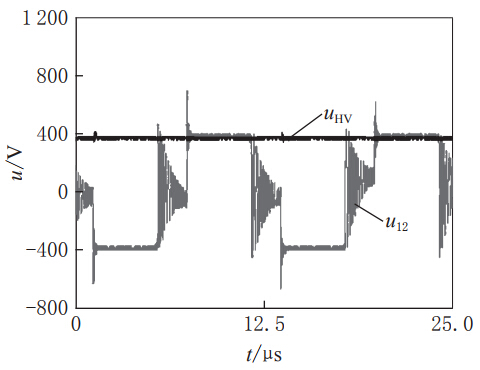Figure 10 The voltage waveform in Boost mode operation

Figure 11 is a schematic diagram of the hardware platform which is mainly composed of main circuit board, control circuit board and corresponding test equipment.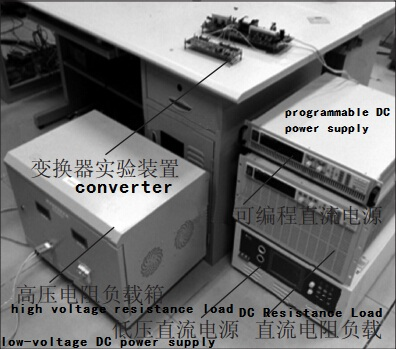Figure 11 physical photographs of experimental devices

4 Epilogue

According to the characteristics of bidirectional DC/DC converter applied in fields of battery and electric vehicle energy storage management, a bidirectional DC/DC converter based on full-bridge push-pull topology is obtained by comparing with different circuit topologies. Different from the traditional state-space average modeling, the method of PWM-switch modeling is used now to build small signal equivalent circuits in different mode operations, and the voltage loop has also been designed and corrected. The high-power constant voltage charging is realized in Buck mode moderation and the high-power constant voltage discharge is also realized in boost mode operation. Both modes can work normally and stably to meet the performance requirements of practical applications, which appear to be in high value at fields of battery and electric vehicle energy storage management.

Book Suggestion

Soft-Switching PWM Full-Bridge Converters: Topologies, Control, and DesignJun 23, 2014

This book intends to describe systematically the soft-switching techniques for pulse-width modulation (PWM) full-bridge converters, including the topologies, control and design, and it reveals the relationship among the various topologies and PWM strategies previously proposed by other researchers. The book not only presents theoretical analysis, but also gives many detailed design examples of the converters.

---by Xinbo Ruan

Power Electronics: Converters and RegulatorsOct 28, 2016

This book is the result of the extensive experience the authors gained through their year-long occupation at the Faculty of Electrical Engineering at the University of Banja Luka. Starting at the fundamental basics of electrical engineering, the book guides the reader into this field and covers all the relevant types of converters and regulators. Understanding is enhanced by the given examples, exercises and solutions. Thus this book can be used as a textbook for students, for self-study or as a reference book for professionals.

---by Branko L. Dokić and Branko Blanuša

Pulse-Width Modulated DC-DC Power ConvertersOct 26, 2015

With improved end-of-chapter summaries of key concepts, review questions, problems and answers, biographies and case studies, this is an essential textbook for graduate and senior undergraduate students in electrical engineering. Its superior readability and clarity of explanations also makes it a key reference for practicing engineers and research scientists. Following the success of Pulse-Width Modulated DC-DC Power Converters this second edition has been thoroughly revised and expanded to cover the latest challenges and advances in the field.

---by Marian K. Kazimierczuk

Relevant information "Modeling and Control of Full Bridge Push-Pull Bi-Directional DC/DC Converter"

About the article "Modeling and Control of Full Bridge Push-Pull Bi-Directional DC/DC Converter", If you have better ideas, don't hesitate to  write your thoughts in the following comment area.

You also can find more articles about electronic semiconductor through Google search engine, or refer to the following related articles:

How to Learn Analog Circuit Design

Look Forward to the Future of Semiconductor

### Related Posts

How to purchase
Feature
After-sales Service